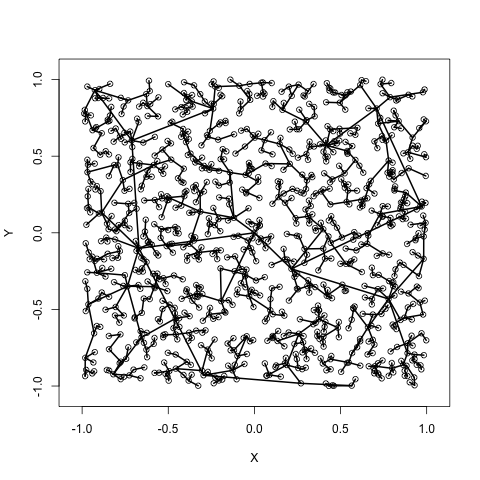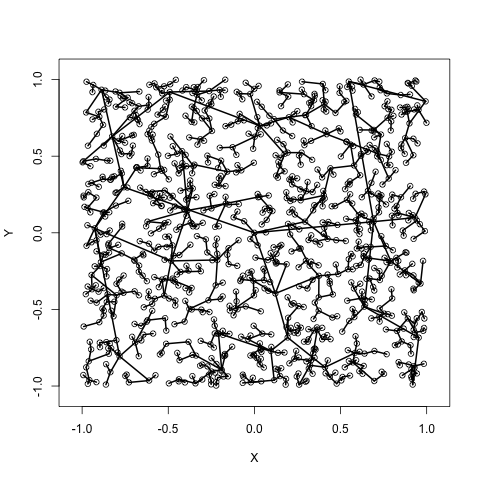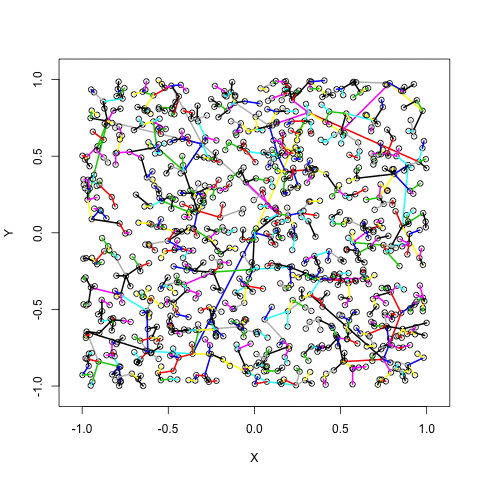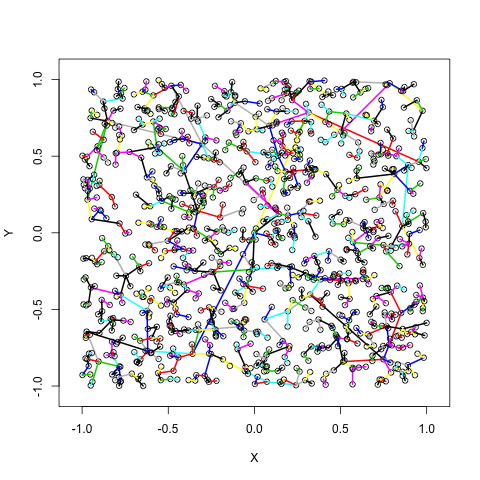# Simulations at a Bar

Published:

I remember when I first entered the mathematics department, I did it specifically because I saw just how excited the professors were about their field. Those first few months were obviously not super exciting as I hadn’t really done any math since high school, and at the time, I had no interest in it nor any interest in continuing it.

Fast forward to last night where I’m up at 1 AM at a bar writing a simulation for fun. My friends and I had just finished a great round of trivia (3rd place and a correct sports question!) when Matt asked

“What would happen if we generated points uniformly and had them connect to their nearest neighbor?”

After much consideration with one arguing that it would be a cycle and two arguing that it would generate a tree, we decided to simulate it and just see what happens! So, the code to generate this random graph is as follows:

X <- c(); X <- 0
Y <- c(); Y <- 0
plot(X, Y, xlim = c(-1.05,1.05), ylim = c(-1.05,1.05))
for (i in 2:1000) {
X[i] <- runif(1,-1,1)
Y[i] <- runif(1,-1,1)
points(X[i], Y[i])
min = 10000
for (j in 1:length(X)) {

if (i != j) {
dist_j <- dist(X[i], Y[i], X[j], Y[j])
if (dist_j < min) {
min <- dist_j
min_x <- X[j]
min_y <- Y[j]
}
}
}
segments(X[i], Y[i], min_x, min_y, lwd = 2)
}


Excuse the brute force, but post-trivia isn’t always the time for optimization. So, we observe the below, which is a .gif of 20 different randomly generate trees (Take that Joe!) as well as one static example for easy of viewing.

Twenty ExamplesOne ExampleOnce we get here, we could stop, look, and say, “Yep. That’s a tree.” Instead, we start to question different properties of it like

1. What is the average number of leaves if we consider the tree starting at the origin?
2. What is the expected depth of a tree?
3. For fun, what if we add colors to the edges! (Maybe these are weights? Maybe it just looks cool?)

The first two questions are definitely solvable, but not by what is now two guys at a bar in one evening that both want to go to sleep. That being said, we still added colors for fun.

Twenty ExamplesOne ExampleI made this post today because it was an exciting moment. It was a random question that we wanted to approach, and it’s those questions that remind me why I love my field.

Tags: